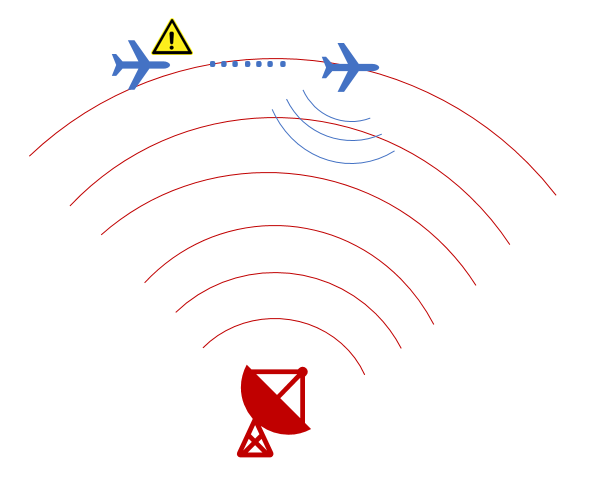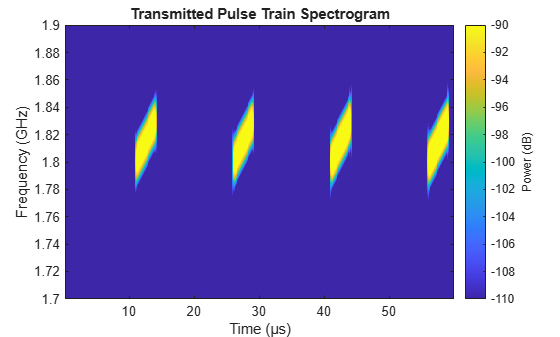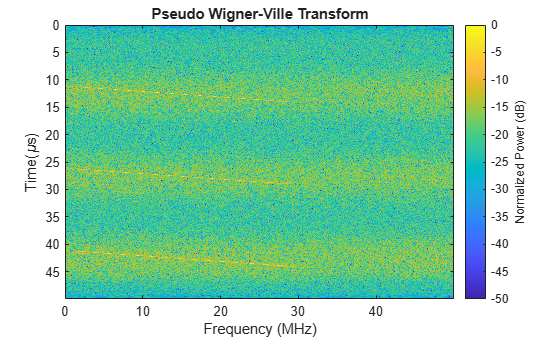Documentation

### This is machine translation

Mouseover text to see original. Click the button below to return to the English version of the page.

Modern aircraft often carry a radar warning receiver (RWR) with them. The RWR detects the radar emission and warns the pilot when the radar signal shines on the aircraft. An RWR can not only detect the radar emission, but also analyze the intercepted signal and catalog what kind of radar is the signal coming from. This example shows how an RWR can estimate the parameters of intercepted signal. The example simulates a radar scenario for a ground surveillance radar (emitter) and a flying aircraft (target) equipped with an RWR. The RWR intercepts radar signals, extracts the waveform parameters from the intercepted signal, and estimates the location of the emitter. The extracted parameters can be utilized by the aircraft to take counter-measures.

This example requires Image Processing Toolbox™

### Introduction

An RWR is a passive electronic warfare support system  that provides timely information to the pilot about its RF signal environment. The RWR intercepts an impinging signal, and uses signal processing techniques to extract information about the intercepted waveform characteristics, as well as the location of the emitter. This information can be used to invoke counter-measures, such as jamming to avoid being detected by the radar. The interaction between the radar and the aircraft is depicted in the following diagram.In this example, we simulate a scenario where a ground surveillance radar and an airplane with an RWR present. The RWR detects the radar signal and extracts the following waveform parameters from the intercepted signal:

1. Pulse repetition interval

2. Center frequency

3. Bandwidth

4. Pulse duration

5. Direction of arrival

6. Position of the emitter

The RWR chain consists of a phased array antenna, a channelized receiver, an envelope detector, and a signal processor. The frequency band of the intercepted signal is estimated by the channelized receiver and the envelope detector, following which the detected sub-banded signal is fed to the signal processor. Beam steering is applied towards the direction of arrival of this sub-banded signal, and the waveform parameters are estimated using pseudo Wigner-Ville transform in conjunction with Hough transform. Using angle of arrival and single-baseline approach, the location of the emitter is also estimated.

### Scenario Setup

Assume the ground based surveillance radar operates in the L band, and transmits chirp signals of 3 $\mu s$ duration at a pulse repetition interval of 15 $\mu s$. Bandwidth of the transmitted chirp is 30 MHz, and the carrier frequency is 1.8 GHz. The surveillance radar is located at the origin and is stationary, and the aircraft is flying at a constant speed of 200 m/s (~0.6 Mach).

% Define the transmitted waveform parameters fs = 4e9; % Sampling frequency for the systems (Hz) fc = 1.8e9; % Operating frequency of the surveillance radar (Hz) T = 3e-6; % Chirp duration (s) PRF = 1/(15e-6); % Pulse repetition frequency (Hz) BW = 30e6; % Chirp bandwidth (Hz) c= physconst('LightSpeed'); % Speed of light in air (m/s) % Assume the surveillance radar is at the origin and is stationary radarPos= [0;0;0]; % Radar position (m) radarVel= [0;0;0]; % Radar speed (m/s) % Assume aircraft is moving with constant velocity rwrPos= [-3000;1000;1000]; % Aircraft position (m) rwrVel= [200; 0; 0]; % Aircraft speed (m/s) % Configure objects to model ground radar and aircraft's relative motion rwrPose = phased.Platform(rwrPos, rwrVel); radarPose = phased.Platform(radarPos, radarVel);

The transmit antenna of the radar is a 8x8 uniform rectangular phased array, having a spacing of $\lambda$/2 between its elements. The signal propagates from the radar to the aircraft and is intercepted and analyzed by the RWR. For simplicity, the waveform is chosen as a linear FM waveform with a peak power of 100 W.

% Configure the LFM waveform using the waveform parameters defined above wavGen= phased.LinearFMWaveform('SampleRate',fs,'PulseWidth',T,'SweepBandwidth',BW,'PRF',PRF); % Configure the Uniform Rectangular Array antennaTx = phased.URA('ElementSpacing',repmat((c/fc)/2, 1, 2), 'Size', [8,8]); % Configure objects for transmitting and propagating the radar signal tx = phased.Transmitter('Gain', 5, 'PeakPower',100); radiator = phased.Radiator( 'Sensor', antennaTx, 'OperatingFrequency', fc); envIn = phased.FreeSpace('TwoWayPropagation',false,'SampleRate', fs,'OperatingFrequency',fc);

The ground surveillance radar is unaware of the direction of the target, therefore, it needs to scan the entire space to look for aircraft. In general, the radar will transmit a series of pulses at each direction before moving to the next direction. Therefore, without losing generality, this example assumes that the radar is transmitting toward zero degrees azimuth and elevation. The following figure shows the time frequency representation of a 4-pulse train arrived at the aircraft. Note that although the pulse train arrives at a specific delay, the time delay of the arrival of the first pulse is irrelevant for the RWR because it has no knowledge transmit time and has to constantly monitor its environment

% Transmit a train of pulses numPulses = 4; txPulseTrain = helperRWR('simulateTransmission',numPulses, wavGen, rwrPos,... radarPos, rwrVel, radarVel, rwrPose, radarPose, tx, radiator, envIn,fs,fc,PRF); % Observe the signal arriving at the RWR pspectrum(txPulseTrain,fs,'spectrogram','FrequencyLimits',[1.7e9 1.9e9], 'Leakage',0.65) title('Transmitted pulse train spectrogram'); caxis([-110 -90]);The RWR is equipped with a 10x10 uniform rectangular array with a spacing of $\lambda$/2 between its elements. It operates in the entire L-band, with a center frequency of 2 GHz. The RWR listens to the environment, and continuously feeds the collected data into the processing chain.

% Configure the receive antenna dip = phased.IsotropicAntennaElement('BackBaffled',true); antennaRx = phased.URA('ElementSpacing',repmat((c/2e9)/2,1,2),'Size', [10,10],'Element',dip); % Model the radar receiver chain collector = phased.Collector('Sensor', antennaRx,'OperatingFrequency',fc); rx = phased.ReceiverPreamp('Gain',0,'NoiseMethod','Noise power', 'NoisePower',2.5e-6,'SeedSource','Property', 'Seed',2018); % Collect the waves at the receiver [~, tgtAng] = rangeangle(radarPos,rwrPos); yr = collector(txPulseTrain,tgtAng); yr = rx(yr);

### RWR envelope detector

The envelope detector in the RWR is responsible for detecting the presence of any signal. As the RWR is continuously receiving data, the receiver chain buffers and truncates the received data into 50 $\mu s$ segments.

% Truncate the received data truncTime = 50e-6; truncInd = round(truncTime*fs); yr = yr(1:truncInd,:);

Since the RWR has no knowledge about the exact center frequency used in the transmit waveform, it first uses a bank of filters, each tuned to a slightly different RF center frequency, to divide the received data into subbands. Then the envelope detector is applied in each band to check whether a signal presents. In this example, the signal is divided into sub-bands of 100 MHz bandwidth. An added benefit for such operation is that instead of sampling the entire bandwidth covered by the RWR, the signal in each subband can be down-sampled to a sampling frequency of 100 MHz.

% Define the bandwidth of each frequency sub-band stepFreq = 100e6; % Calculate number of sub-bands and configure dsp.Channelizer numChan = fs/stepFreq; channelizer = dsp.Channelizer('NumFrequencyBands', numChan, 'StopbandAttenuation', 80);

The plot below shows the first four band created by the filter bank.

% Visualize the first four filters created in the filter bank of the % channelizer freqz(channelizer, 1:4) title('Zoomed Channelizer response for first four filters') xlim([0 0.2])% Pass the received data through the channelizer subData = channelizer(yr);

The received data, subData, has 3 dimensions. The first dimension represents the fast-time, the second dimension represents the sub-bands, and the third dimension corresponds to the receiving elements of the receiving array. For the RWR's 10x10 antenna configuration used in this example, we have 100 receiving elements. Because the transmit power is low and the receiver noise is high, the radar signal is indistinguishable from the noise. Therefore the received power are summed across these elements to enhance the signal-to-nose ratio (SNR) and get a better estimates of the power in each subband. The band that has the maximum power is the one used by the radar.

% Rearrange the subData to combine the antenna array channels only incohsubData = pulsint(permute(subData,[1,3,2]),'noncoherent'); incohsubData = squeeze(incohsubData); % Plot power distribution subbandPow = pow2db(rms(incohsubData,1).^2)+30; plot(subbandPow); xlabel('Band Index'); ylabel('Power (dBm)');% Find the sub-band with maximum power [~,detInd] = max(subbandPow); 

### RWR signal processor

Although the power in the selected band is higher compared to the neighboring band, the SNR within the band is still low, as shown in the following figure.

subData = (subData(:,detInd,:)); subData = squeeze(subData); %adjust the data to 2-D matrix % Visualize the detected sub-band data plot(mag2db(abs(sum(subData,2)))+30) ylabel('Power (dBm)') title('Detected sub-band from 100 channels combined incoherently')% Find the original starting frequency of the sub-band having the detected % signal detfBand = fs*(detInd-1)/(fs/stepFreq); % Update the sampling frequency to the decimated frequency fs = stepFreq;

The subData is now a two-dimensional matrix. The first dimension represents fast-time samples and the second dimension is the data across 100 receiving antenna channels. The detected sub-band starting frequency is calculated to find the carrier frequency of the detected signal.

The next step for the RWR is to find the direction from which the radio waves are arriving. This angle of arrival information would be used to steer the receive antenna beam in the direction of the emitter, and locate the emitter on the ground using single base-line approach. The RWR estimates the direction of arrival using a two dimensional MUSIC estimator. Beam steering is done using phase-shift beamformer to achieve maximum SNR of the signal, thus help the waveform parameter extraction.

Assume that ground plane is flat and parallel to the xy-plane of the coordinate system. such, the RWR can use the altitude information from its altimeter readings of the aircraft along with the direction of arrival to triangulate the location of the emitter.

% Configure the MUSIC Estimator to find the direction of arrival of the % signal doaEst = phased.MUSICEstimator2D('OperatingFrequency',fc,'PropagationSpeed',c,... 'SensorArray',antennaRx,'DOAOutputPort',true,'AzimuthScanAngles',-50:.5:50,... 'ElevationScanAngles',-50:.5:50, 'NumSignalsSource', 'Property','NumSignals', 1); [mSpec,doa] = doaEst(subData); plotSpectrum(doaEst,'Title','2-D MUSIC Spatial Spectrum Top view'); view(0,90); axis([-30 0 -30 0]);The figure clearly shows the location of the emitter.

% Configure the beamformer object to steer the beam before combining the % channels beamformer = phased.PhaseShiftBeamformer('SensorArray',antennaRx,... 'OperatingFrequency',fc,'DirectionSource','Input port'); % Apply the beamforming, and visualize the beam steered radiation % pattern mBeamf = beamformer(subData, doa); % Find the location of the emitter altimeterElev = rwrPos(3); d = abs(altimeterElev/sind(doa(2)));

After applying the beam steering, the antenna has the maximum gain in the azimuth and elevation angle of arrival of the signal. This further improves the SNR of the intercepted signal. Next, the signal parameters are extracted in the signal processor using one of the time-frequency analysis techniques known as pseudo Wigner-Ville transform coupled with Hough transform as described in .

First, derive the time frequency representation of the intercepted signal using Wigner-Ville transform.

% Compute the pseudo Wigner-Ville transform [tpwv,t,f] = helperRWR('pWignerVille',mBeamf,fs); % Plot the pseudo Wigner-Ville transform imagesc(f*1e-6,t*1e6,pow2db(abs(tpwv./max(tpwv(:))))); xlabel('Frequency (MHz)'); ylabel('Time(\mus)'); caxis([-50 0]); clb = colorbar; clb.Label.String = 'Normalized Power (dB)'; title ('Pseudo Wigner-Ville Transform')Using human eyes, even though the resulting time frequency representation is noisy, it is not too hard to separate the signal from the background. Each pulse appears as a line in the time frequency plane. Thus, using beginning and end of the time-frequency lines, we can derive the pulse width and the bandwidth of the pulse. Similarly, the time between lines from different pulses gives us the pulse repetition interval.

To do this automatically without relying on human eyes, we use Hough transform to identify those lines from the image. The Hough transform can perform well in the presence of noise, and is an enhancement to the time-frequency signal analysis method.

To use Hough transform, it is necessary to convert the time frequency image into a binary image. Next code snippet performs some data smoothing on the image and then use imbinarize to do the conversion. The conversion threshold can be modified based on the signal-noise characteristics of the receiver and the operating environment.

% Normalize the pseudo Wigner-Ville image twvNorm = abs(tpwv)./max(abs(tpwv(:))); % Implement a median filter to clear the noise filImag = medfilt2(twvNorm,[7 7]); % Use threshold to convert filtered image into binary image BW = imbinarize(filImag./max(filImag(:)), 0.15); imagesc(f*1e-6,t*1e6,BW); colormap('gray'); xlabel('Frequency (MHz)'); ylabel('Time(\mus)'); title ('Pseudo Wigner-Ville Transform - BW')Using Hough transform, the binary pseudo Wigner-Ville image are first transformed to peaks. This way, instead of detecting the line in an image, we just need to detect a peak in an image.

% Compute the Hough transform of the image and plot [H,T,R] = hough(BW); imshow(H,[],'XData',T,'YData',R,'InitialMagnification','fit'); xlabel('\theta'), ylabel('\rho'); axis on, axis normal, hold on; title('Hough transform of the image')

The peak positions are extracted using houghpeaks.

% Compute peaks in the transform, up to 5 peaks P = houghpeaks(H,5); x = T(P(:,2)); y = R(P(:,1)); plot(x,y,'s','color','g'); xlim([-90 -50]); ylim([-5000 0])Using these positions, houghlines can reconstruct the lines in the original binary image. Then as discussed earlier, the beginning and the end of these lines help us estimate the waveform parameters.

lines = houghlines(BW,T,R,P,'FillGap',3e-6*fs,'MinLength',1e-6*fs); coord = [lines(:).point1; lines(:).point2]; % Plot the detected lines superimposed on the binary image clf; imagesc(f*1e-6, t*1e6, BW); colormap(gray); hold on xlabel('Frequency (MHz)') ylabel('Time(\mus)') title('Hough transform - detected lines') for ii = 1:2:2*size(lines,2) plot(f(coord(:,ii))*1e-6, t(coord(:,ii+1))*1e6,'LineWidth',2,'Color','green'); end% Calculate the parameters using the line co-ordinates pulDur = t(coord(2,2)) - t(coord(1,2)); % Pulse duration bWidth = f(coord(2,1)) - f(coord(1,1)); % Pulse Bandwidth pulRI = abs(t(coord(1,4)) - t(coord(1,2))); % Pulse repetition interval detFc = detfBand + f(coord(2,1)); % Center frequency

The extracted waveform characteristics are listed below. They match the truth very well. These estimates can then be used to catalog the radar and prepare for counter measures if necessary.

helperRWR('displayParameters',pulRI, pulDur, bWidth,detFc, doa,d);
Pulse repetition interval = 14.97 microseconds Pulse duration = 2.84 microseconds Pulse bandwidth = 27 MHz Center frequency = 1.8286 GHz Azimuth angle of emitter = -18.5 degrees Elevation angle of emitter = -17.5 degrees Distance of the emitter = 3325.5095 m 

### Summary

This demo shows how an RWR can estimate the parameters of the intercepted radar signal using signal processing and image processing techniques.

### References

 Electronic Warfare and Radar Systems Engineering Handbook 2013, Naval Air Warfare Center Weapons Division, Point Mugu, California.

 Daniel L. Stevens, Stephanie A. Schuckers, Detection and Parameter Extraction of Low Probability of Intercept Radar Signals using the Hough Transform . Global Journal of Research In Engineering Vol 15 Issue 6, Jan. 2016